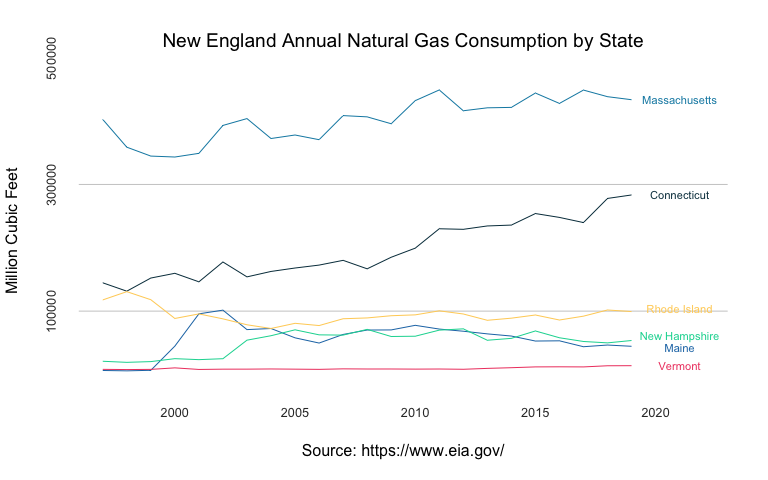The USgas package provides an overview of demand for natural gas in the US in a time-series format. That includes the following datasets:

• us_total - The US annual natural gas consumption by state-level between 1997 and 2019, and aggregate level between 1949 and 2019
• us_monthly - The monthly demand for natural gas in the US between 2001 and 2020
• us_residential - The US monthly natural gas residential consumption by state and aggregate level between 1989 and 2020

Data source: The US Energy Information Administration API

More information about the package datasets available on this vignette.

## Installation

You can install the released version of USgas from CRAN with:

install.packages("USgas")


And the development version from GitHub with:

# install.packages("devtools")
devtools::install_github("RamiKrispin/USgas")


## Example

Plotting the consumption of natural gas in New England states:

data(us_total)

str(us_total)
#> 'data.frame':    1266 obs. of  3 variables:
#>  $year : int 1997 1998 1999 2000 2001 2002 2003 2004 2005 2006 ... #>$ state: chr  "Alabama" "Alabama" "Alabama" "Alabama" ...
#>  $y : int 324158 329134 337270 353614 332693 379343 350345 382367 353156 391093 ... head(us_total) #> year state y #> 1 1997 Alabama 324158 #> 2 1998 Alabama 329134 #> 3 1999 Alabama 337270 #> 4 2000 Alabama 353614 #> 5 2001 Alabama 332693 #> 6 2002 Alabama 379343  Subsetting the New England states: ne <- c("Connecticut", "Maine", "Massachusetts", "New Hampshire", "Rhode Island", "Vermont") ne_gas <- us_total[which(us_total$state %in% ne),]

ne_wide <- reshape(ne_gas, v.names = "y", idvar = "year",
timevar = "state", direction = "wide")
ne_wide <- ne_wide[order(ne_wide$year), ] names(ne_wide) <- c("year",ne) head(ne_wide) #> year Connecticut Maine Massachusetts New Hampshire Rhode Island Vermont #> 139 1997 144708 6290 402629 20848 117707 8061 #> 140 1998 131497 5716 358846 19127 130751 7735 #> 141 1999 152237 6572 344790 20313 118001 8033 #> 142 2000 159712 44779 343314 24950 88419 10426 #> 143 2001 146278 95733 349103 23398 95607 7919 #> 144 2002 177587 101536 393194 24901 87805 8367  Plotting the states series: # Set the y and x axis ticks at_x <- seq(from = 2000, to = 2020, by = 5) at_y <- pretty(ne_gas$y)[c(2, 4, 6)]

# plot the first series
plot(ne_wide$year, ne_wide$Connecticut,
type = "l",
col = "#073b4c",
frame.plot = FALSE,
axes = FALSE,
panel.first = abline(h = c(at_y), col = "grey80"),
main = "New England Annual Natural Gas Consumption by State",
cex.main = 1.2, font.main = 1, col.main = "black",
xlab = "Source: https://www.eia.gov/",
font.axis = 1, cex.lab= 1,
ylab = "Million Cubic Feet",
ylim = c(min(ne_gas$y, na.rm = TRUE), max(ne_gas$y, na.rm = TRUE)),
xlim = c(min(ne_gas$year), max(ne_gas$year) + 3))

# Add the 5 other series
lines(ne_wide$year, ne_wide$Maine, col = "#1f77b4")
lines(ne_wide$year, ne_wide$Massachusetts, col = "#118ab2")
lines(ne_wide$year, ne_wide$New Hampshire, col = "#06d6a0")
lines(ne_wide$year, ne_wide$Rhode Island, col = "#ffd166")
lines(ne_wide$year, ne_wide$Vermont, col = "#ef476f")

# Add the y and x axis ticks

mtext(side =1, text = format(at_x, nsmall=0), at = at_x,
col = "grey20", line = 1, cex = 0.8)

mtext(side =2, text = format(at_y, scientific = FALSE), at = at_y,
col = "grey20", line = 1, cex = 0.8)

text(max(ne_wide$year) + 2, tail(ne_wide$Connecticut,1),
"Connecticut",
col = "#073b4c",
cex = 0.7)

text(max(ne_wide$year) + 2, tail(ne_wide$Maine,1) * 0.95,
"Maine",
col = "#1f77b4",
cex = 0.7)

text(max(ne_wide$year) + 2, tail(ne_wide$Massachusetts,1),
"Massachusetts",
col = "#118ab2",
cex = 0.7)

text(max(ne_wide$year) + 2, tail(ne_wide$New Hampshire,1) * 1.1,
"New Hampshire",
col = "#06d6a0",
cex = 0.7)

text(max(ne_wide$year) + 2, tail(ne_wide$Rhode Island,1) * 1.05,
"Rhode Island",
col = "#ffd166",
cex = 0.7)

text(max(ne_wide$year) + 2, tail(ne_wide$Vermont,1),
"Vermont",
col = "#ef476f",
cex = 0.7)